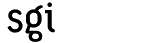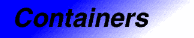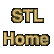# hash_multimap<Key, Data, HashFcn, EqualKey, Alloc>Category: containers Component type: type

### Description

Hash_multimap is a Hashed Associative Container that associates objects of type Key with objects of type Data. Hash_multimap is a Pair Associative Container, meaning that its value type is pair<const Key, Data>. It is also a Multiple Associative Container, meaning that there is no limit on the number of elements whose keys may compare equal using EqualKey.

Looking up an element in a hash_multimap by its key is efficient, so hash_multimap is useful for "dictionaries" where the order of elements is irrelevant. If it is important for the elements to be in a particular order, however, then multimap is more appropriate.

### Example

```struct eqstr
{
bool operator()(const char* s1, const char* s2) const
{
return strcmp(s1, s2) == 0;
}
};

typedef hash_multimap<const char*, int, hash<const char*>, eqstr> map_type;

void lookup(const map_type& Map, const char* str)
{
cout << str << ": ";
pair<map_type::const_iterator, map_type::const_iterator> p =
Map.equal_range(str);
for (map_type::const_iterator i = p.first; i != p.second; ++i)
cout << (*i).second << " ";
cout << endl;
}

int main()
{
map_type M;
M.insert(map_type::value_type("H", 1));
M.insert(map_type::value_type("H", 2));
M.insert(map_type::value_type("C", 12));
M.insert(map_type::value_type("C", 13));
M.insert(map_type::value_type("O", 16));
M.insert(map_type::value_type("O", 17));
M.insert(map_type::value_type("O", 18));
M.insert(map_type::value_type("I", 127));

lookup(M, "I");
lookup(M, "O");
lookup(M, "Rn");
}
```

### Definition

Defined in the header hash_map, and in the backward-compatibility header hash_map.h. This class is an SGI extension; it is not part of the C++ standard.

### Template parameters

Parameter Description Default
Key The hash_multimap's key type. This is also defined as hash_multimap::key_type.
Data The hash_multimap's data type. This is also defined as hash_multimap::data_type.
HashFcn The hash function used by the hash_multimap. This is also defined as hash_multimap::hasher. hash<Key>
EqualKey The hash_multimap's key equality function: a binary predicate that determines whether two keys are equal. This is also defined as hash_multimap::key_equal. equal_to<Key>
Alloc The hash_set's allocator, used for all internal memory management. alloc

### Model of

Multiple Hashed Associative Container, Pair Associative Container

None.

### Members

Member Where defined Description
key_type Associative Container The hash_multimap's key type, Key.
data_type Pair Associative Container The type of object associated with the keys.
value_type Pair Associative Container The type of object, pair<const key_type, data_type>, stored in the hash_multimap.
hasher Hashed Associative Container The hash_multimap's hash function.
key_equal Hashed Associative Container Function object that compares keys for equality.
pointer Container Pointer to T.
reference Container Reference to T
const_reference Container Const reference to T
size_type Container An unsigned integral type.
difference_type Container A signed integral type.
iterator Container Iterator used to iterate through a hash_multimap. 
const_iterator Container Const iterator used to iterate through a hash_multimap.
iterator begin() Container Returns an iterator pointing to the beginning of the hash_multimap.
iterator end() Container Returns an iterator pointing to the end of the hash_multimap.
const_iterator begin() const Container Returns an const_iterator pointing to the beginning of the hash_multimap.
const_iterator end() const Container Returns an const_iterator pointing to the end of the hash_multimap.
size_type size() const Container Returns the size of the hash_multimap.
size_type max_size() const Container Returns the largest possible size of the hash_multimap.
bool empty() const Container true if the hash_multimap's size is 0.
size_type bucket_count() const Hashed Associative Container Returns the number of buckets used by the hash_multimap.
void resize(size_type n) Hashed Associative Container Increases the bucket count to at least n.
hasher hash_funct() const Hashed Associative Container Returns the hasher object used by the hash_multimap.
key_equal key_eq() const Hashed Associative Container Returns the key_equal object used by the hash_multimap.
hash_multimap() Container Creates an empty hash_multimap.
hash_multimap(size_type n) Hashed Associative Container Creates an empty hash_multimap with at least n buckets.
```hash_multimap(size_type n,
const hasher& h)
```
Hashed Associative Container Creates an empty hash_multimap with at least n buckets, using h as the hash function.
```hash_multimap(size_type n,
const hasher& h,
const key_equal& k)
```
Hashed Associative Container Creates an empty hash_multimap with at least n buckets, using h as the hash function and k as the key equal function.
```template <class InputIterator>
hash_multimap(InputIterator, InputIterator)

```
Multiple Hashed Associative Container Creates a hash_multimap with a copy of a range.
```template <class InputIterator>
hash_multimap(InputIterator, InputIterator,
size_type n)

```
Multiple Hashed Associative Container Creates a hash_multimap with a copy of a range and a bucket count of at least n.
```template <class InputIterator>
hash_multimap(InputIterator, InputIterator,
size_type n, const hasher& h)

```
Multiple Hashed Associative Container Creates a hash_multimap with a copy of a range and a bucket count of at least n, using h as the hash function.
```template <class InputIterator>
hash_multimap(InputIterator, InputIterator,
size_type n, const hasher& h,
const key_equal& k)

```
Multiple Hashed Associative Container Creates a hash_multimap with a copy of a range and a bucket count of at least n, using h as the hash function and k as the key equal function.
hash_multimap(const hash_multimap&) Container The copy constructor.
hash_multimap& operator=(const hash_multimap&) Container The assignment operator
void swap(hash_multimap&) Container Swaps the contents of two hash_multimaps.
iterator insert(const value_type& x) Multiple Associative Container Inserts x into the hash_multimap.
```template <class InputIterator>
void insert(InputIterator, InputIterator)

```
Multiple Associative Container Inserts a range into the hash_multimap.
void erase(iterator pos) Associative Container Erases the element pointed to by pos.
size_type erase(const key_type& k) Associative Container Erases the element whose key is k.
void erase(iterator first, iterator last) Associative Container Erases all elements in a range.
void clear() Associative Container Erases all of the elements.
const_iterator find(const key_type& k) const Associative Container Finds an element whose key is k.
iterator find(const key_type& k) Associative Container Finds an element whose key is k.
size_type count(const key_type& k) const Associative Container Counts the number of elements whose key is k.
```pair<const_iterator, const_iterator>
equal_range(const key_type& k) const
```
Associative Container Finds a range containing all elements whose key is k.
```pair<iterator, iterator>
equal_range(const key_type& k)
```
Associative Container Finds a range containing all elements whose key is k.
```bool operator==(const hash_multimap&,
const hash_multimap&)
```
Hashed Associative Container Tests two hash_multimaps for equality. This is a global function, not a member function.

### New members

All of hash_multimap's members are defined in the Multiple Hashed Associative Container and Pair Associative Container requirements. Hash_multimap does not introduce any new members.

### Notes

 Hash_multimap::iterator is not a mutable iterator, because hash_multimap::value_type is not Assignable. That is, if i is of type hash_multimap::iterator and p is of type hash_multimap::value_type, then *i = p is not a valid expression. However, hash_multimap::iterator isn't a constant iterator either, because it can be used to modify the object that it points to. Using the same notation as above, (*i).second = p is a valid expression.

 This member function relies on member template functions, which at present (early 1998) are not supported by all compilers. If your compiler supports member templates, you can call this function with any type of input iterator. If your compiler does not yet support member templates, though, then the arguments must either be of type const value_type* or of type hash_multimap::const_iterator.